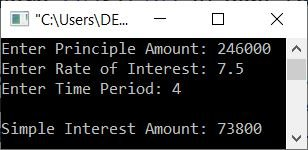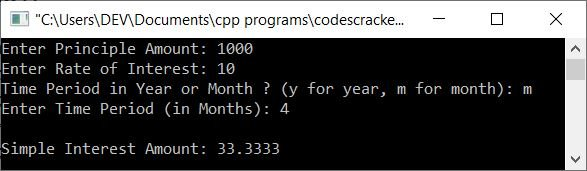# C++ Program to Calculate Simple Interest

This article contains some programs in C++ that are used to find, calculate, and print simple interest based on the data entered by the user at run-time. The following information must be entered by the user at run-time:

• principle amount: amount of money that has to be deposited or borrowed.
• rate of interest: the interest rate that has to be paid or received in return.
• time period: for how many years has the money been deposited or borrowed?

If you're getting confused about the above data, then forget that and look at the formula for simple interest, given below, and then its program. After reading all the things provided here, you'll know everything about the program.

#### Formula to calculate simple interest

The formula to find simple interest is:

`SI = (P*R*T)/100`

where SI stands for simple interest, P stands for the principle amount (deposit or loan amount), R stands for the rate of interest, and T stands for the time period (in years).

## Calculate Simple Interest in C++

The question is, "Write a program in C++ to find and print simple interest based on the P, R, and T (data) entered by the user at run-time." The program given below is the answer to this question:

```#include<iostream>

using namespace std;
int main()
{
float p, r, t, si;
cout<<"Enter Principle Amount: ";
cin>>p;
cout<<"Enter Rate of Interest: ";
cin>>r;
cout<<"Enter Time Period: ";
cin>>t;
si = (p*r*t)/100;
cout<<"\nSimple Interest Amount: "<<si;
cout<<endl;
return 0;
}```

The following snapshot is taken from the initial output of the above C++ program for calculating simple interest:Now enter the data, say 246,000 as the principle amount, 7.5 as the rate of interest, and 4 as the time period. Hit the ENTER key to calculate and print the simple interest amount as shown in the snapshot given below:The calculation of simple interest based on P = 246000, R = 7.5, and T = 4 goes in this way:

```SI = (P*R*T)/100
= (246000*7.5*4)/100
= (246000*30)/100
= (7380000)/100
= 73800```

That is, you'll get the return of \$73,800 if you have deposited the amount; otherwise, you'll have to pay \$73,800 if you've borrowed the loan from an individual, a bank, or any other source.

Note: Enter the time period in years only. If you want to go for six months, then enter 6/12, which is 0.5 year.

For a more interactive and good user experience program, you can also modify the above program in a way that the user gets options for whether to enter the time period in years or months, as shown in the program given below:

```#include<iostream>

using namespace std;
int main()
{
float p, r, t, si;
char ch;
cout<<"Enter Principle Amount: ";
cin>>p;
cout<<"Enter Rate of Interest: ";
cin>>r;
cout<<"Time Period in Year or Month ? (y for year, m for month): ";
cin>>ch;
if(ch=='y')
{
cout<<"Enter Time Period (in Years): ";
cin>>t;
si = (p*r*t)/100;
}
else
{
cout<<"Enter Time Period (in Months): ";
cin>>t;
t = t/12;
si = (p*r*t)/100;
}
cout<<"\nSimple Interest Amount: "<<si;
cout<<endl;
return 0;
}```

Here is its sample run with 1000 as the principle amount, 10 as the rate of interest, and m as the choice (for months as the time period), and finally 4 as the total number of months as the time period:## Using the function, compute simple interest

Let's create the same program, that is, calculating simple interest, but instead of using the normal process, use a user-defined function named find_si(). This function takes three arguments for p (principal amount), r (rate of interest), and t (time period):

```#include<iostream>

using namespace std;

float find_si(float, float, float);
int main()
{
float p, r, t, si;
cout<<"Enter Principle Amount: ";
cin>>p;
cout<<"Enter Rate of Interest: ";
cin>>r;
cout<<"Enter Time Period: ";
cin>>t;
si = find_si(p, r, t);
cout<<"\nSimple Interest Amount: "<<si;
cout<<endl;
return 0;
}
float find_si(float a, float b, float c)
{
float si;
si = (a*b*c)/100;
return si;
}```

This program generates similar results to the previous program. The only difference is that this program was created using a user-defined function.

## Calculate simple interest using the class

This is the last program created using classes. That is, the CodesCracker class is created.Inside the class, I've created a private variable named res and a public function named find_si(). Now, using the class, I've defined the function named find_si(), as done in the previous program.

Inside the main() method, I've created an object of the class CodesCracker. So that, using the object cc_obj, I can access the member function (find_si()) of the class (CodesCracker):

```#include<iostream>

using namespace std;

class CodesCracker
{
private:
int res;
public:
float find_si(float, float, float);
};
float CodesCracker::find_si(float a, float b, float c)
{
res = (a*b*c)/100;
return res;
}

int main()
{
float p, r, t, si;
CodesCracker cc_obj;

cout<<"Enter Principle Amount: ";
cin>>p;
cout<<"Enter Rate of Interest: ";
cin>>r;
cout<<"Enter Time Period: ";
cin>>t;

si = cc_obj.find_si(p, r, t);
cout<<"\nSimple Interest Amount: "<<si;
cout<<endl;
return 0;
}```

This program generates output that is similar to the output of the first program.

C++ Quiz

« Previous Program Next Program »